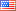##### Stretching algorithm for global scheduling of real-time DAG tasks
Publication Type
Conference abstract/paper published in a peer review journal
Authors

Parallelism is becoming more important nowadays due to the increasing use of multiprocessor systems. A Directed Acyclic Graph (DAG) is a general model of parallel tasks with inter-subtask parallelism. It consists of a collection of dependent subtasks under precedence constraints. In this paper, we study the problem of scheduling n periodic parallel real-time DAG tasks on m homogeneous multiprocessor systems. The dependencies between subtasks make scheduling process more challenging. We propose a stretching algorithm to be applied on each DAG task prior to scheduling process. Thus, DAGs are transformed into a set of independent sequential threads with intermediate offsets and deadlines. The threads obtained due to the transformation are of two types, (i) fully-stretched master threads with utilization equal to 1 and (ii) independent constrained-deadline threads. We propose a scheduling method over RTOS to ensure the execution of fully-stretched master threads on dedicated processors while the remaining processors $$\overline{m} \le m$$, are used to schedule the independent constrained-deadline threads using any multiprocessor scheduling algorithm. In this work, we analyze the stretching algorithm while considering two global preemptive scheduling algorithms from different priority assignment families; the Global Earliest Deadline First (GEDF) from the fixed job priority family, and the Global Deadline Monotonic (GDM) from the fixed task priority family. We prove that GEDF scheduling of stretched threads has a resource augmentation bound equal to $$\frac{3+\sqrt{5}}{2}$$ for all task sets with $$n < \varphi \times \overline{m}$$, where n is the number of tasks in the set and $$\varphi$$ is the golden ratio (the value of the golden ratio is $$\frac{1+\sqrt{5}}{2}$$). While GDM has a resource augmentation bound equal to $$2+\sqrt{3}$$ for all task sets with $$n < \frac{1+\sqrt{3}}{2} \times \overline{m}$$.

Journal
Title
Real-Time Systems
Publisher
Springer
Publisher Country
United States of AmericaIndexing
Scopus
Impact Factor
None
Publication Type
Both (Printed and Online)
Volume
--
Year
2018
Pages
1-31